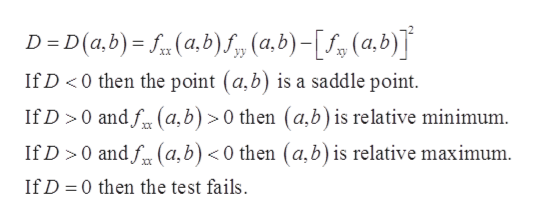# Find the critical points for the function:f(x,y)=x3+y3-9x2-48y-3and use the Second Derivative Test to classify each as a local maximum, local minimum, saddle point, or none of these.

Question

Find the critical points for the function:

f(x,y)=x3+y3-9x2-48y-3

and use the Second Derivative Test to classify each as a local maximum, local minimum, saddle point, or none of these.

check_circleExpert Solution
Step 1

To determine the critical points for the function and classify the critical points.

Step 2

Given:

Step 3

Concept Used:

The critical points of the function f(x,y) satisfy the condition fx = 0 and fy = 0  or fx and/or fy does not exist.

Calculate the discrim...help_outlineImage Transcriptionclose(a,b) S,, (a,b)-(a.b) D D(a,b) XX If D <0 then the point (a,b) is a saddle point If D 0 andf(a,b) 0 then (a,b) is relative minimum If D 0 andf(a,b) < 0 then (a,b) is relative maximum Xx If D 0 then the test fails fullscreen

### Want to see the full answer?

See Solution

#### Want to see this answer and more?

Solutions are written by subject experts who are available 24/7. Questions are typically answered within 1 hour*

See Solution
*Response times may vary by subject and question
Tagged in

### Functions